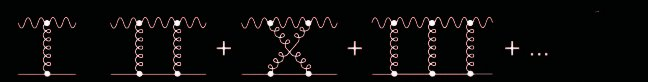# Observational constraints to ‘fat graviton’ theories

Raman Sundrum and others have postulated that the seemingly arbitrary milli-eV energy scale implied by the phenomenon of cosmic acceleration could be explained if gravity is a low energy effective field theory in which gravitons cease to gravitate past some critical momentum. Robert Caldwell and I showed that if the cutoff scale scale corresponds to a milli-eV, existing x-ray lenses wouldn’t be possible.

Results published in astro-ph/0606133 (Phys. Rev. Lett. 100, 031301).

To do this, we did a tree-level calculation (first pair of diagrams immediately below) of the cross-section for gravitational lensing (analagous to Rutherford scattering) in linearized quantum gravity with a cutoff, and then extended the calculation to all orders in perturbation theory (series of diagrams below the first pair). This calculation only converges if we restrict ourselves to the eikonal (geometric-optics) limit, which certainly applies in the astrophysical scenarios of interest.

##Such a theory can be derived from a Lagrangian density non-linear in the d’Alembertian.

We also show below the x-ray lensing contours of the lensing system Q0957+661 provided to us by George Chartas for our analysis: